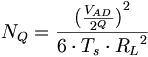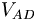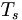# Quantization noise

During the quantization process some precision gets lost, this changes the signal somewhat, the difference between the original signal and the quantized signal is called quantization noise or the (rounding error). It is commonly expressed by the root-mean-square error equation in engineering.$N_Q = { { { ( { {V_{AD_{\,\!}}} \over 2^Q } ) } ^2} \over {6 \cdot T_s \cdot {R_L}^2} }$$Q~$ is the resolution or the amount of bits in the converter$V_{AD}~$ is the analog voltage range of converter expressed in (Volts)$T_s~$ is the sampling interval of the converter expressed in (Seconds)${R_L}^2$ is resistance of the converter expressed in (Ohms)